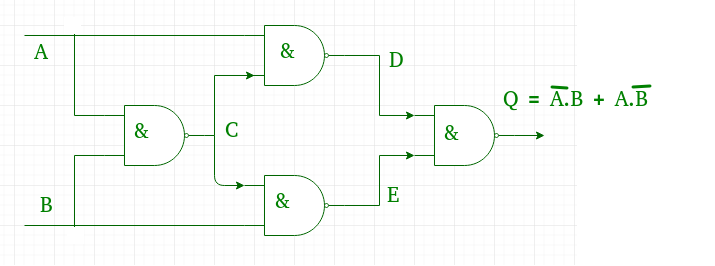# GATE | GATE-IT-2004 | Question 8

What is the minimum number of NAND gates required to implement a 2-input EXCLUSIVE-OR function without using any other logic gate?
(A) 3
(B) 4
(C) 5
(D) 6

Explanation:Pic Courtesy: http://www.electronics-tutorials.ws/logic/logic_7.html

Other way around:

x XOR y = x’y+xy’ = x’y+xy’+xx’+yy’ = (x+y) (x’+y’)

Using NAND gates

F= (x+y)(xy)’ = x. (xy)’ + y. (xy)’

Taking compliment

F’= ( x. (xy)’ + y. (xy)’ )’ = (x. (xy)’)’. (y. (xy)’)

Compliment again

F=( (x. (xy)’)’. (y. (xy)’) )’